# LOOPS IN JAVA FOR FOREACH WHILE

Loops In Java | Core Java Tutorial | Minigranth

# Loops in Java : Introduction

• Loops are the essential part of any programming language, such as loops in java. If you have to repeat (iterate) some statements multiple times (say you have to print count up to 1000 ) then we use loops.
• We can write either 1000 out.print() statements which will print counting from 1-1000 ( that will be a real headache) or we can use loops to make it easy.
• Loops in java exponentially decrease the effort, memory and number of line of code allowing better readability of the code.
• There exists four different types of loops in java which are used according to the need and requirement within the logic.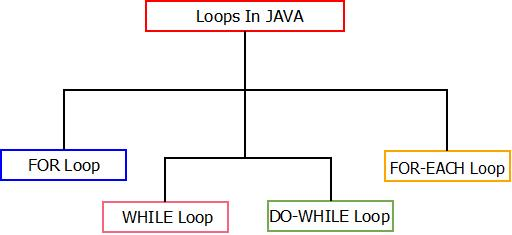Loops In Java : Types

• We will be discussing all of these loops in java, one by one in detail along with their respective syntaxes and examples for each of them.

### Loops In Java : The Discussion

1. #### Loops In Java : The For Loop

• This is the most simple for loops in java which iterate some statements in program several time based on some conditions. Below is the mentioned syntax, flowchart and example for it.

Syntax
 for(initialization;test-condition;increment/decrement) {           //statements to be iterated; }

FlowchartThe For Loop : Flowchart

Example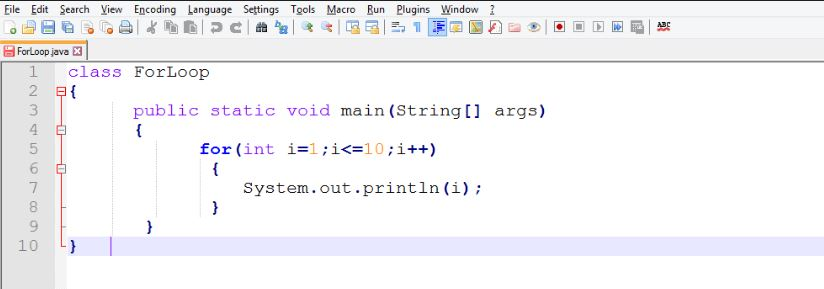The For Loop : Example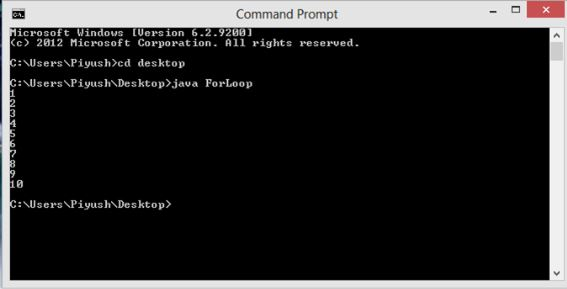The For Loop : Output

##### When to Use For-Loop?
• We use for loop when we know how many times we have to iterate a particular part of a program.

2. #### Loops In Java : The While Loop

• This is another type of loop which do the same i.e iterate statements in a part of a program. Below is the mentioned syntax, flowchart and example for it.

Syntax
 while(condition) {           //statements to be iterated;           increment/decrement counter; }

FlowchartThe While Loop : Flowchart

Example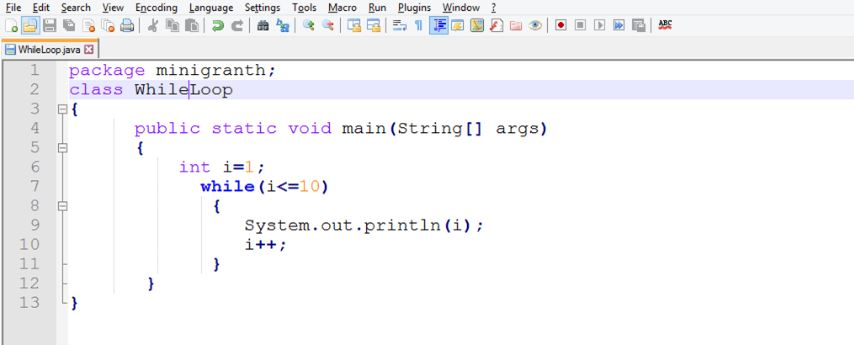The While Loop : Example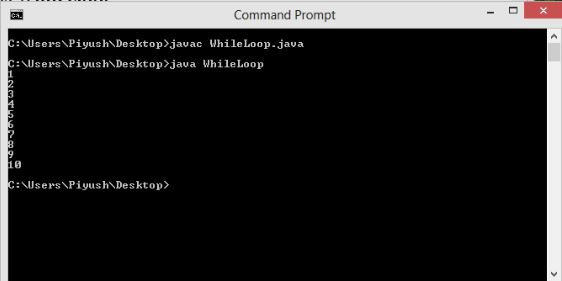The While Loop : Output

 Note : While loop is a entry controlled loop because it check for the condition before entering the block. If the condition is true loop executes else loop exits.

##### When to use While-Loop?
• While loop is used when we don’t know how many times we have to iterate a part of the program i.e the number of iterations are not fixed.

3. #### Loops In Java : The do-While Loop

• This is a exit controlled loop which check for the condition after the set of statements are executed. It also iterates statements in part of the program.

Syntax
 do {           //statements to be iterated;           increment/decrement counter; }while(condition);

FlowchartThe Do-While Loop : Flowchart

Example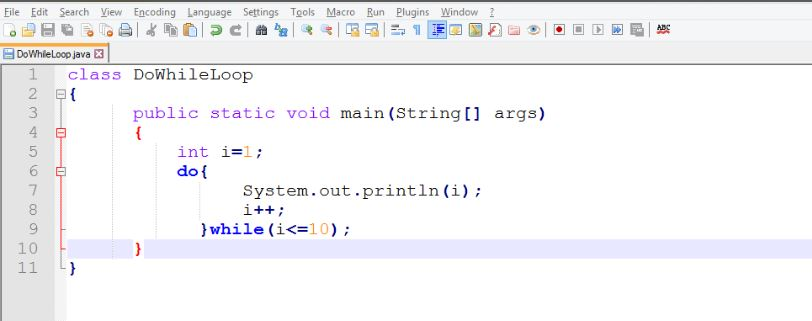The Do-While Loop : Example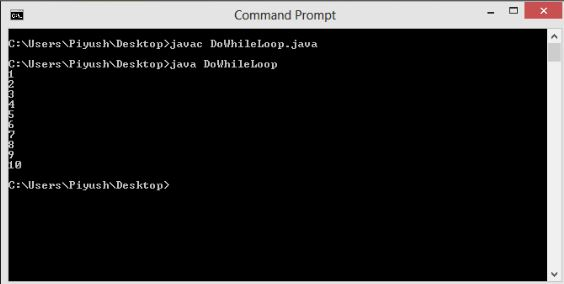The Do-While Loop : Output

##### When to use do-While Loop?
• We use do-while loop when we want to execute a part of a program once even if condition is false. Since do-while loop check for condition after executing the loop block so the block is executed once even if condition is false.

 Note : You have to be careful in specifying condition for loops . If you specify wrong conditions the loop will run infinitely and the program will crash. For example : for(;;) , while(true){} ,do{}while(true);

4. ### Loops In Java : The For-Each Loop

• For-each loop is a new type of loop introduced in Java.
• It is generally used to traverse array as we do in other loops.
• Also, For-each loop differ from other loops as you don’t have to declare and initialize a loop counter variable. In this we declare a variable  of same type as of array type.
• For-each loop do not maintain index, hence we can’t modify the content of array.

Syntax
 foreach (type variable : array) {             //statements; }

ExampleThe For-Each Loop : Example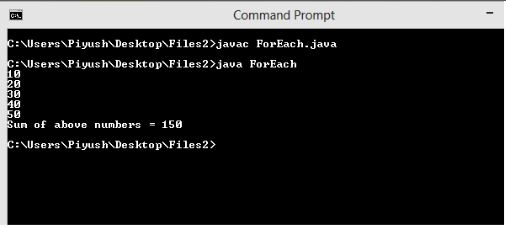The For-Each Loop : Output